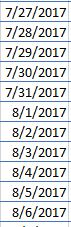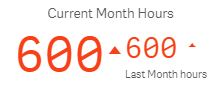# New to Qlik Sense

Discussion board where members can get started with Qlik Sense.

HighlightedNot applicable

## Need Help with Syntax

Hi,

I have a problem in which I am trying to show the comparison between current month's total work hours logged by employee vs the last month. (I am using KPI for this purpose)

My table has date as shown, all sequentially:I basically want to sum the total hours for the last month and current month and show that as a KPI.

I tried Sum( {“>=\$(=Min(Date))<=\$(=Max(Date))”} } EmpHrs) but its not giving any result.

Any inputs shall be greatly appreciated.

Thanks

Tags (3)
1 Solution

Accepted SolutionsPartner

## Re: Need Help with Syntax

Current Month Hours = =sum(   {<Date = {">=\$(=MonthStart(Max(Date))) <=\$(=MonthEnd(Max(Date))) "}>}     EmpHrs)

Previous Month Hours = =sum(   {<Date = {">=\$(=MonthStart(Max(Date),-1)) <=\$(=MonthEnd(Max(Date),-1)) "}>}     EmpHrs)

7 RepliesMVP

## Re: Need Help with Syntax

Try this ways

=sum(   {<Date = {">=\$(=Min(Date)) <=\$(=Max(Date)) "}>}     EmpHrs)

Regards

AnandNot applicable

## Re: Need Help with Syntax

Hi Anand,

This works but how do I get the hours for the next month.

As in If I use the same formula for Current month it gives me the same value. I have used this right now in last month's formula.

I wanted something like this:ThanksPartner

## Re: Need Help with Syntax

Current Month Hours = =sum(   {<Date = {">=\$(=MonthStart(Max(Date))) <=\$(=MonthEnd(Max(Date))) "}>}     EmpHrs)

Previous Month Hours = =sum(   {<Date = {">=\$(=MonthStart(Max(Date),-1)) <=\$(=MonthEnd(Max(Date),-1)) "}>}     EmpHrs)MVP

## Re: Need Help with Syntax

Ex:-

Num(Month(Datefield)) as NumMonth

From Location;

Then in expression for current month

=sum(  {<Date = {">=\$(=Min(Date)) <=\$(=Max(Date)) "}, NumMonth = {"\$(=Num(Month(MAX(Date ))))"} >}  EmpHrs)

And for previous month

=sum(  {<Date = {">=\$(=Min(Date)) <=\$(=Max(Date)) "}, NumMonth = {"\$(=Num(Month(MAX(Date )))-1) "} >}  EmpHrs)Not applicable

## Re: Need Help with Syntax

Thanks Chris.

This worked well.Not applicable

## Re: Need Help with Syntax

Thank you for the response Anand.

This also worked for me.MVP